# 西藏地区积雪深度模型分析与研究Analysis and Research on the Model of Snow Depth in Tibet Area

DOI: 10.12677/CCRL.2019.82027, PDF, HTML, XML, 下载: 394  浏览: 566

Abstract: In this paper, by using 35-year daily observation data of ground, it establishes model for snow depth in Tibet. By the use of impact factor such as daily precipitation, average temperature, the lowest temperature, the highest temperature, snow depth, numerical forecast precipitation of the next day, and average temperature, it establishes multiple regression equations that predict snow depth of the next day. Due to the lack of numerical forecasting data, it uses the observed data of the next day instead of numerical forecasting data. It selects five sites in which daily average amount of snow is largest to draw scatter diagram, and obtain correlation coefficient (R) and standard deviation (SEE) to test. Then it establishes the seasonal model of snow depth by improving method and analyses. Comparing imitative effect before and after improvement, it shows that required correlation coefficient (R) of equal has increased and standard deviation (SEE) has decreased after improving. All of the above descriptions’ mean analog value after improving method is much closer to actual value.

1. 引言

2. 资料与方法

2.1. 数据介绍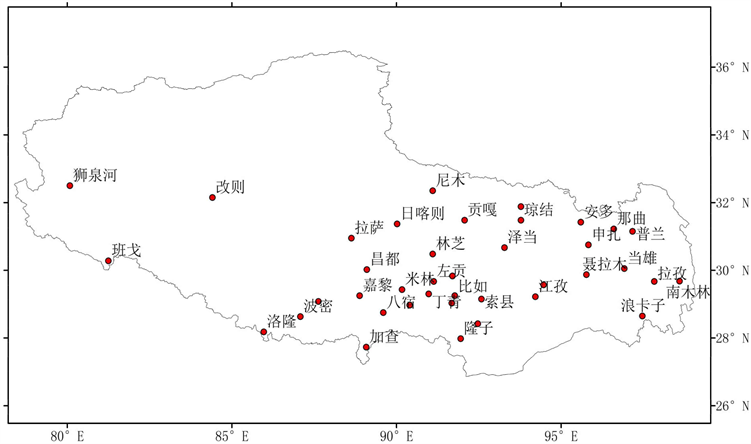Figure 1. Distribution of stations in the study area

2.2. 研究方法Table 1. Basic information of the station in the study area

3. 结果与讨论

3.1. 积雪模型建立

$y^={b}_{0}+{b}_{1}{x}_{1}+{b}_{2}{x}_{2}+{b}_{3}{x}_{3}+{b}_{4}{x}_{4}+{b}_{5}{x}_{5}+{b}_{6}{x}_{6}+{b}_{7}{x}_{7}$ (6)Table 2. Coefficient table of snow model

Continued

3.2. 积雪模型检验Table 3. Coefficient table of snow model at five stations in which daily average amount of snow is largest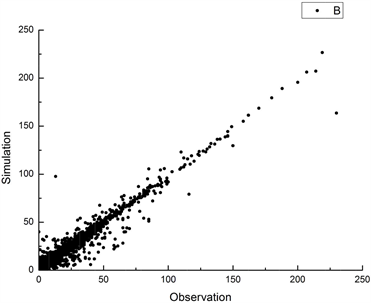(a) 聂拉木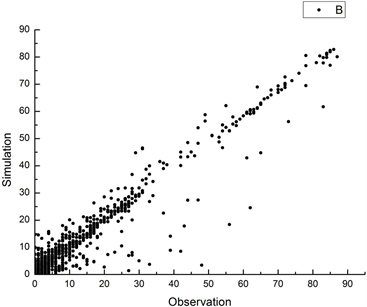(b) 帕里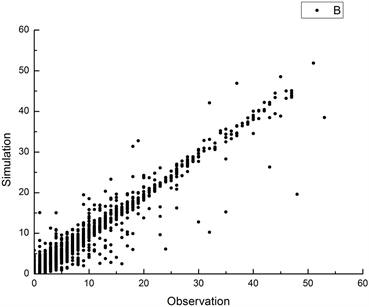(c) 普兰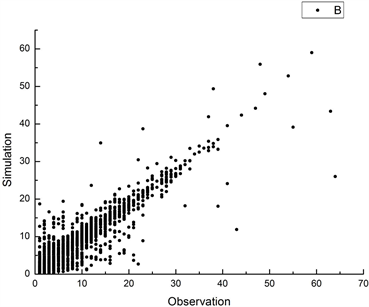(d) 错那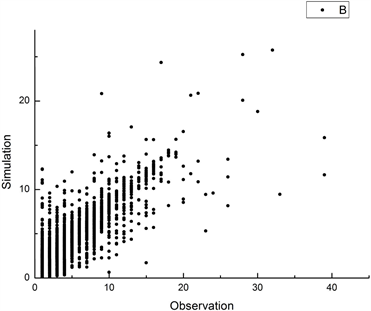(e) 嘉黎

Figure 2. Test chart of scattered points at five stations in which daily average amount of snow is largest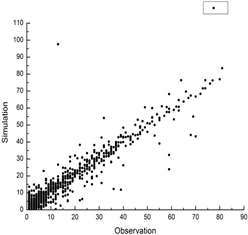(a) 春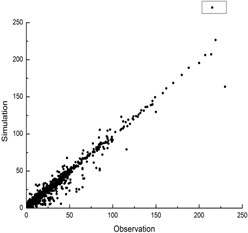(a) 秋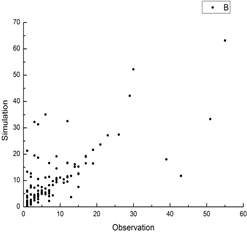(a) 冬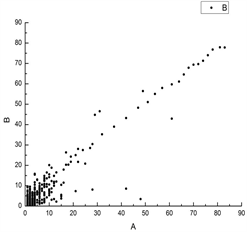(b) 春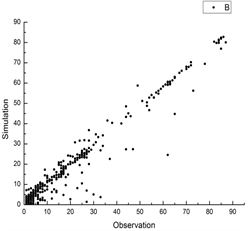(b) 秋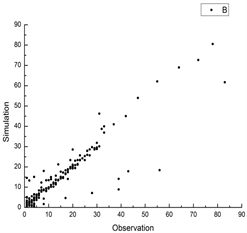(b) 冬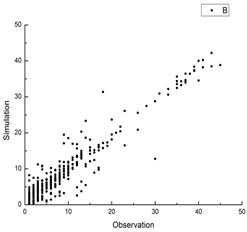(c) 春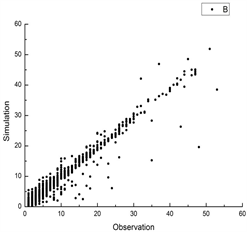(c) 秋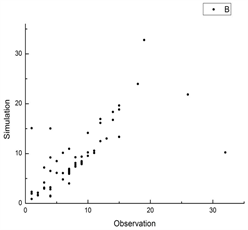(c) 冬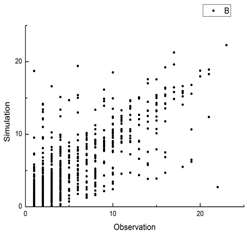(d) 春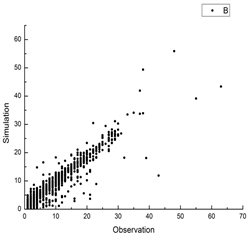(d) 秋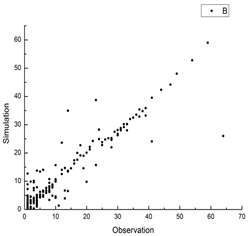(d) 冬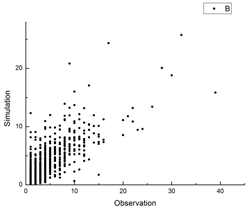(e) 春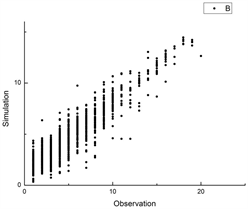(e) 秋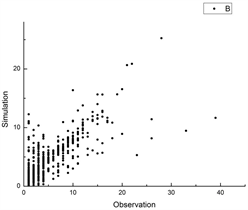(e) 冬

Figure 3. Seasonal test chart of scattered points at five stations in which daily average amount of snow is largest ((a) Nielamu; (b) Pali; (c) Pulan; (d) Cuona; (e) Jiali)Table 4. Basic test parameters at five stations in which daily average amount of snow is largestTable 5. Seasonal test of correlation coefficient (R) at five stations in which daily average amount of snow is largest

3.3. 积雪模型改进Table 6. Seasonal coefficient table of snow depth model at five stations in which daily average amount of snow is largest

Continued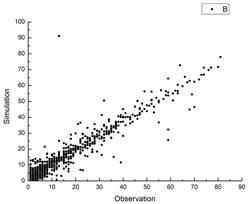(a) 春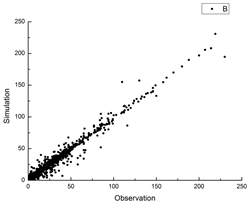(a) 秋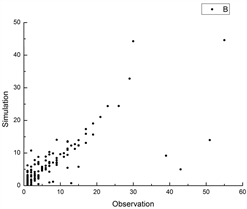(a) 冬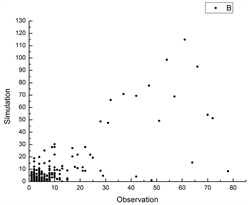(b) 春(b) 秋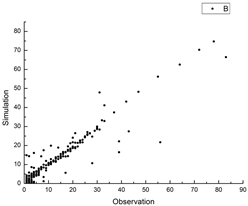(b) 冬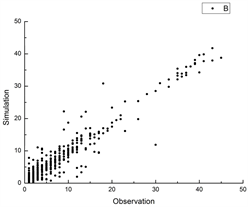(c) 春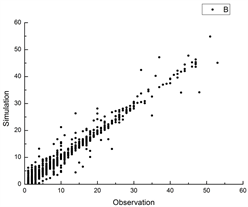(c) 秋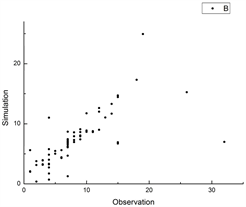(c) 冬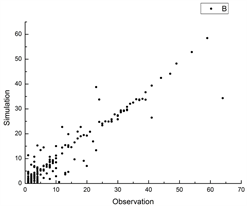(d) 春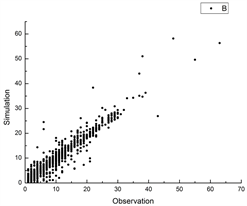(d) 秋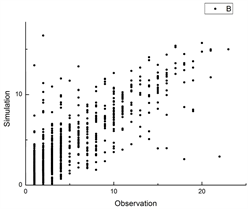(d) 冬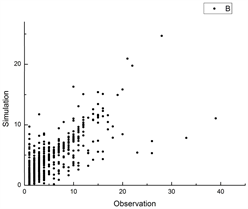(e) 春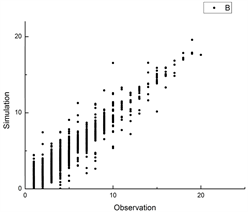(e) 秋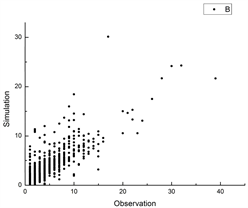(e) 冬

Figure 4. Improved seasonal test chart of scattered points at five stations in which daily average amount of snow is largest ((a) Nielamu; (b) Pali; (c) Pulan; (d) Cuona; (e) Jiali)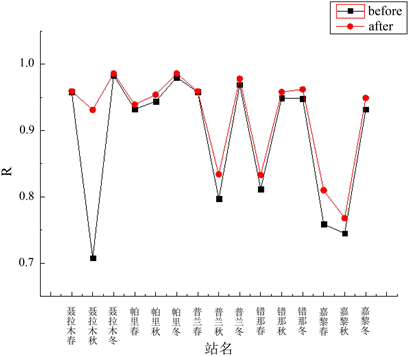Figure 5. Comparison of correlation coefficients (R) before/after improvementTable 7. Comparison of correlation coefficients (R) before/after improvementTable 8. Comparison of standard deviation (SEE) before/after improvement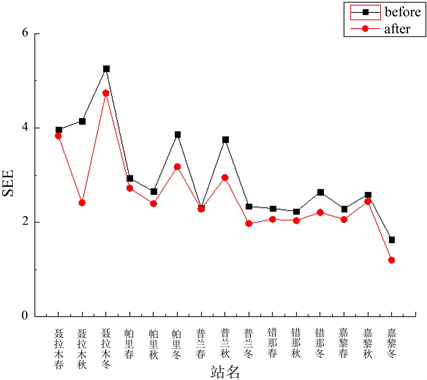Figure 6. Comparison of standard deviation (SEE) before/after improvement

4. 结论

1) 对积雪量不同的台站分别建立模型，可以发现积雪量较大的台站相关系数明显高于积雪量较小的台站，并且积雪量较大的台站拟合效果好于积雪量较小的台站。例如日平均积雪深度最大的聂拉木站的散点图拟合效果最好，而日平均积雪最小的嘉黎站的拟合效果最差。

2) 温度和降水分季节对积雪深度的影响不同，分季节检验后发现拟合值和观测值有较大偏差，说明用同一个方程来分析不同季节的拟合效果并不科学。所以在建立积雪深度模型时不能采用一个观测站建一个模型的方法，要分季节建立模型进行讨论才比较具有可靠性。

  白淑英, 史建桥, 高吉喜, 卜军. 1979-2010年青藏高原积雪深度时空变化遥感分析[J]. 地球信息科学学报, 2014, 16(4): 628-637.  王澄海, 王芝兰, 崔洋. 40余年来中国地区季节性积雪的空间分布及年际变化特征[J]. 冰川冻土, 2009, 31(2): 301-310.  王叶堂, 何勇, 侯书贵. 2000-2005年青藏高原积雪时空变化分析[J]. 冰川冻土, 2007, 29(6): 855-861.  马丽娟, 秦大河. 1957-2009年中国台站观测的关键积雪参数时空变化特征[J]. 冰川冻土, 2012, 34(1): 1-11.  邹志伟. 西藏雪灾对高原牧业的影响探析(1824-1957) [J]. 西北大学学报(自然科学版), 2011, 41(6): 1059-1063.  巴桑, 杨秀海, 拉珍, 郑照军, 旷达, 拉巴. 基于多源数据的西藏地区积雪变化趋势分析[J]. 冰川冻土, 2012, 34(5): 1023-1030.  韦志刚, 黄荣辉, 陈文, 董文杰. 青藏高原地面站积雪的空间分布和年代际变化特征[J]. 大气科学, 2002, 26(4): 496-508.  白淑英, 史建桥, 高吉喜, 卜军. 1979-2010年青藏高原积雪深度时空变化遥感分析[J]. 地球信息科学学报, 2014, 16(4): 628-637.  王叶堂, 何勇, 侯书贵. 2000-2005年青藏高原积雪时空变化分析[J]. 冰川冻土, 2007, 29(6): 855-861.